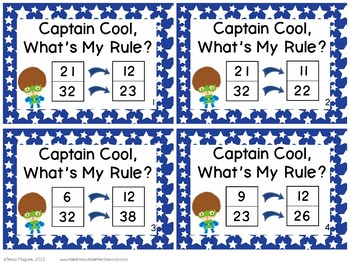# Function Rules in Addition & Subtraction: Captain Cool, What's your Rule?

Rated 4.91 out of 5, based on 38 reviews
38 Ratings;
1st - 2nd, Homeschool
Subjects
Resource Type
Standards
Formats Included
• PDF
Pages
38 pages
Report this resource to TPT

### Description

3 different centers practicing applying functions with input & outut tables (also known as function machines). Function rules are a crucial skill in the upper grades, and applying them in addition and subtraction help build that foundation with primary grades students. Functions can also help students build their understanding of the inverse relationship between addition and subtraction. This Captain Cool, What's My Rule unit will have students working to achieve their missions while identifying rules, applying rules, and generating equations following rules.

There are directions pages for student use as well as recording sheets for each center. Each center is set up as a different superhero mission, with the student (Captain Cool) completing each mission to rescue his helicopter, super car, and sidekick. There's also certificate when students have completed each challenge in the mission and completed the centers.

Center Directions

Center 1: Students roll a dice and then add or subtract that number based on what is on their card to fill in the out table. Students complete two cards. There is a blank template for you to create your own, as well.

Center 2: Students draw a card and determine the rule. There are two different problems with the same rule on each card. Students record the two addends and the rule on their recording sheet for both problems. Students complete 5 cards.

Center 3: The rule is on these cards. You can have students self-generate numbers for their in/out boxes, or you can have students roll the dice to get the first number for the table. They continue until they’ have filled out the table with 5 different numbers, and then students solve a second card.

Total Pages
38 pages
N/A
Teaching Duration
N/A
Report this resource to TPT
Reported resources will be reviewed by our team. Report this resource to let us know if this resource violates TPT’s content guidelines.

### Standards

to see state-specific standards (only available in the US).
Add within 100, including adding a two-digit number and a one-digit number, and adding a two-digit number and a multiple of 10, using concrete models or drawings and strategies based on place value, properties of operations, and/or the relationship between addition and subtraction; relate the strategy to a written method and explain the reasoning used. Understand that in adding two-digit numbers, one adds tens and tens, ones and ones; and sometimes it is necessary to compose a ten.
Fluently add and subtract within 100 using strategies based on place value, properties of operations, and/or the relationship between addition and subtraction.
Add and subtract within 1000, using concrete models or drawings and strategies based on place value, properties of operations, and/or the relationship between addition and subtraction; relate the strategy to a written method. Understand that in adding or subtracting three-digit numbers, one adds or subtracts hundreds and hundreds, tens and tens, ones and ones; and sometimes it is necessary to compose or decompose tens or hundreds.
Add and subtract within 20, demonstrating fluency for addition and subtraction within 10. Use strategies such as counting on; making ten (e.g., 8 + 6 = 8 + 2 + 4 = 10 + 4 = 14); decomposing a number leading to a ten (e.g., 13 - 4 = 13 - 3 - 1 = 10 - 1 = 9); using the relationship between addition and subtraction (e.g., knowing that 8 + 4 = 12, one knows 12 - 8 = 4); and creating equivalent but easier or known sums (e.g., adding 6 + 7 by creating the known equivalent 6 + 6 + 1 = 12 + 1 = 13).
Determine the unknown whole number in an addition or subtraction equation relating three whole numbers. For example, determine the unknown number that makes the equation true in each of the equations 8 + ? = 11, 5 = ▯ - 3, 6 + 6 = ▯.